How to Learn in 24 Hours?

 Need Help? M-F: 9am-5pm(PST): Toll-Free: (877) RAPID-10 or 1-877-727-4310 24/7 Online Technical Support: The Rapid Support Center vip@rapidlearningcenter.com Secure Online Order:Need Proof? Testimonials by Our Users

 Rapid Learning Courses: MCAT in 24 Hours (2021-22) USMLE in 24 Hours (Boards) Chemistry in 24 Hours Biology in 24 Hours Physics in 24 Hours Mathematics in 24 Hours Psychology in 24 Hours SAT in 24 Hours ACT in 24 Hours AP in 24 Hours CLEP in 24 Hours DAT in 24 Hours (Dental) OAT in 24 Hours (Optometry) PCAT in 24 Hours (Pharmacy) Nursing Entrance Exams Certification in 24 Hours eBook - Survival Kits Audiobooks (MP3)

 Tell-A-Friend: Have friends taking science and math courses too? Tell them about our rapid learning system.Home »  Mathematics »  College Algebra

 Topic Review on "Title": Quadratic Equation: A quadratic equation is a specific type of equation written on the standard form: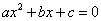where. The Zero Product Rule: If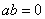, then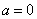orSquare Root Method: Ifand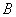are algebraic expressions such that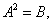then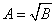orCompleting the Square Method: Completing the square consists of adding to both sides of an equation a positive number that can help factor a quadratic equation. Quadratic Formula: The solution of a quadratic equation of the formdepends on the sign of the discriminant. Quadratic Inequality: A quadratic inequality is a quadratic equation where the equality sign is replaced by an inequality. Quadratic Function: A quadratic function is a function where for eachthe real number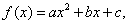a, b, and c are real numbers with a not equal to 0. Parabola: A parabola is the graph of a quadratic function. Vertex: A vertex is the point where the parabola intersects the axis of symmetry.

Rapid Study Kit for "Title":
 Flash Movie Flash Game Flash Card Core Concept Tutorial Problem Solving Drill Review Cheat Sheet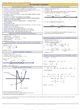"Title" Tutorial Summary : This tutorial describes what a quadratic function is and its properties. The square root method is used to solve quadratic equations. Important principles such as completing the square of a quadratic equation are shown with the use of examples. The quadratic formula and the conditions under which it is used are described in this tutorial. Quadratic Inequalities are defined and how they differ from quadratic equations. Techniques showing how you solve a quadratic inequality with roots are mentioned in this tutorial with the use of examples. Finally, quadratic equations are defined along with the use of their graphs. The vertex and the axis of symmetry are defined with the use graphical examples.

 Tutorial Features: Specific Tutorial Features: • Step by step examples are shown to introduce quadratic equations, square root method, and graphs of quadratic functions. • The quadratic equation and the quadratic formula are shown with the use of examples. Concept questions are asked to test the important ideals of quadratic equations. Series Features: • Concept map showing inter-connections of new concepts in this tutorial and those previously introduced. • Definition slides introduce terms as they are needed. • Visual representation of concepts • Animated examples—worked out step by step • A concise summary is given at the conclusion of the tutorial.